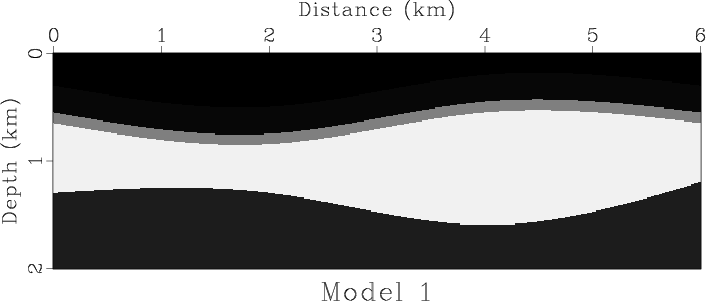sfunif2 creates a layered model from specified interfaces. It is inspired by an analogous program in Seismic Unix.

The following example from rsf/su/rsfmodel shows a simple model generated with sfunif2 and reproduced from an example in the book Seismic Data Processing with Seismic Un*x by David Forel, Thomas Benz, and Wayne Pennington.The input to sfunif2 is a file containing sampled interfaces. The velocities in the layers can be specified as linear distributions
$$v(z,x) = v_0 + v_z\,(z-z_0)+v_x\,(x-x_0)$$
with parameters given by v00=, dvdz=, dvdx=, z0=, and x0=. These parameters should be lists, with the number of elements greater by one than the number of interfaces. The desired sampling of the depth axis is specified by n1=, o1=, and d1= parameters.

The 3-D version is sfunif3. See the following example from rsf/rsf/unif3In addition to specifying layer velocities as linear (constant gradient) distributions, sfunif3 allows these properties to be specified in an optional file.